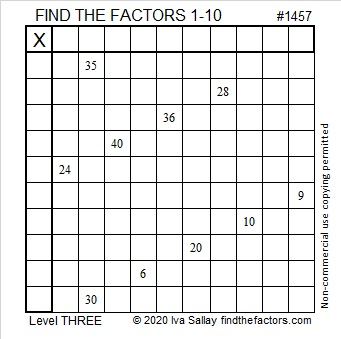# 1457 and Level 3

Contents

### Today’s Puzzle:

What numbers are common factors of 35 and 30? That’s the first question you need to solve this puzzle. All that’s left afterward is to look at the clues in the puzzle from top to bottom and write their factors in the factor column and the factor row. Remember, only use numbers from 1 to 10 as factors.### Factors of 1457:

• 1457 is a composite number.
• Prime factorization: 1457 = 31 × 47
• 1457 has no exponents greater than 1 in its prime factorization, so √1457 cannot be simplified.
• The exponents in the prime factorization are 1, and 1. Adding one to each exponent and multiplying we get (1 + 1)(1 + 1) = 2 × 2 = 4. Therefore 1457 has exactly 4 factors.
• The factors of 1457 are outlined with their factor pair partners in the graphic below.### Facts about the number 1457:

1457 is the difference of two squares in two different ways:
729² – 728² = 1457
39² – 8² = 1457

This site uses Akismet to reduce spam. Learn how your comment data is processed.